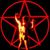# Calculate coordinates of Polyline in ArcGIS online with Arcade

3629
5
05-18-2019 02:28 PMNew Contributor II

How can you calculate the start and stop coordinates of a polyline in ArcGIS online using Arcade?

With points you can use the following expression.

var CoorX = Round(geometry(\$feature).x, 6);

var originShift = 2.0 * PI * 6378137.0 / 2.0;
var lon = (CoorX / originShift) * 180.0;
// var lat = (y / originShift) * 180.0;

// lat = 180.0 / PI * (2.0 * Atan( Exp( lat * PI / 180.0)) - PI / 2.0);
return lon;

What is a comparable expression to use for a polyline feature? I am just trying to get the coordinates at the start and end of the polyline. I don't need any points in between.

Tags (3)
1 Solution

Accepted SolutionsbyEsri Esteemed Contributor

This mentions the way the paths are constructed:

A three-dimensional array of numbers. The inner-most array contains the x,y,z,m coordinates of a single point. The second dimension contains additional points making up a line segment. The third dimension allows the polyline to have multiple segments.

To get to the first and last point you simply can do this (in this case "line" is the line geometry), like in Geometry(\$feature)

``````var paths = line.paths;
var first_point = paths;
var last_point = paths[Count(paths)-1];
return first_point.x + " - " + first_point.y;
//return last_point.x + " - " + last_point.y;‍‍‍‍‍``````

Remember that your view scale will influence the precision of your result.

`Be aware that using \$feature as input to this function will yield results only as precise as the view's scale resolution. Therefore values returned from expressions using this function may change after zooming between scales.`
5 RepliesbyEsri Esteemed Contributor

This mentions the way the paths are constructed:

A three-dimensional array of numbers. The inner-most array contains the x,y,z,m coordinates of a single point. The second dimension contains additional points making up a line segment. The third dimension allows the polyline to have multiple segments.

To get to the first and last point you simply can do this (in this case "line" is the line geometry), like in Geometry(\$feature)

``````var paths = line.paths;
var first_point = paths;
var last_point = paths[Count(paths)-1];
return first_point.x + " - " + first_point.y;
//return last_point.x + " - " + last_point.y;‍‍‍‍‍``````

Remember that your view scale will influence the precision of your result.

`Be aware that using \$feature as input to this function will yield results only as precise as the view's scale resolution. Therefore values returned from expressions using this function may change after zooming between scales.`New Contributor II

Thank you Xander for the solution.

I had to use var last_point = paths[Count(paths)-1]; to get the last point. It didn't like paths for some reason but I got it working. Not sure if other people would have this issue.

Thank you again.byEsri Esteemed Contributor

I did my test on a multipart polyline I assume mine was formed differently. Good catch.New Contributor II

Thank you again for the solution!New Contributor III

For points, THIS worked like a charm. Funny I had to find it in a post that wasn't exactly what I was looking for. That said, what would this look like if I wanted to points to be in WGS 1984 Web Mercator (auxiliary sphere)?